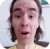FreeKB - Java Programming Understanding variables
Java Programming - Understanding variablesFollowing are examples of different types of variables in Java.

byte

numbers between -128 through 127. Uses less memory than short, int, and long. When a value is not defined, the default value is 0. In this example, 0 will be printed.

``````byte number;
out.println(number);``````

The specific value can be specified. In this example, 123 will be printed.

``````number = 123;
out.println(number);``````

short

numbers between -32768 and 32767. Uses more memory than byte, less memory than int and long. When a value is not defined, the default value is 0. In this example, 0 will be printed.

``````short number;
out.println(number);``````

The specific value can be specified. In this example, 12345 will be printed.

``````number = 12345;
out.println(number);``````

int

large numbers. Uses more memory than byte and short, less memory than long. When a value is not defined, the default value is 0. In this example, 0 will be printed.

``````int number;
out.println(number);``````

The specific value can be specified. In this example, 123456789 will be printed.

``````number = 123456789;
out.println(number);``````

long

very large numbers. Uses more memory than byte, short, and int. When a value is not defined, the default value is 0. In this example, 0 will be printed.

``````long number;
out.println(number);``````

The specific value can be specified. In this example, 1234567890123456789 will be printed.

``````bignumber = 1234567890123456789;
out.println(number);``````

float

floating point numbers. Do not use this for currency. Instead, use the java.math.BigDecimal class. When a value is not defined, the default value is 0.0. In this example, 0.0 will be printed.

``````float number;
out.println(number);``````

The specific value can be specified. In this example, 1.2 will be printed.

``````number = 1.2;
out.println(number);``````

double

floating point numbers. Do not use this for currency. Instead, use the java.math.BigDecimal class. When a value is not defined, the default value is 0.0. In this example, 0.0 will be printed.

``````double number;
out.println(number);``````

The specific value can be specified. In this example, 1.2 will be printed.

``````number = 1.2;
out.println(number);``````

boolean

contain a value of only true or false. The default value is false.

``````boolean result;
if (result == true) {
out.println("result is true");
}
else {
out.println("result is false");
}``````

We will never share your name or email with anyone. Enter your email if you would like to be notified when we respond to your comment.

Please enter af38d in the box below so that we can be sure you are a human.

Web design by yours truely - me, myself, and I   |   jeremy.canfield@freekb.net   |